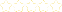+Introduction to Solutions and Molarity

Owner: BRIAN MILLER
Description:
Students will be introduced to the basic concepts of solutions, solutes, and solvents, and the process of calculating concentration through Molarity.

Your Quiz Points in this Playlist

Total Possible
0
2 Tutorials in this Playlist

Solutions Overview

Author:
Rating:(1)
This tutorial has a quiz
•• -

Calculating Molarity

Author:
Rating:(0)
This tutorial has a quiz
•• -